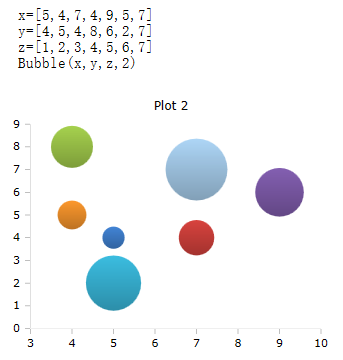# Bubble Function

Drawing bubbles in the plot box

### Description

The $$Bubble$$ function draws bubbles in a plot box. Three lists are expected as parameters, for the X-positions, the Y-positions and the size.

### Syntax

Bubble (list x, list y, list z)

Bubble (list x, list y, list z, id)

### Parameters

Liste x

A list of the X positions of the bubbles.

Liste y

A list of the Y-positions of the bubbles.

Liste z

A list of sizes of bubbles.

The specification of the size z is not an absolute value, but approximately represents the ratio of the values to one another. A z-list with the values 200, 500, and 800 will show bubbles the same size as a list with the values 20, 50, and 80.

### Optional parameters

id

Id number of the plot box, if more than one box is open on the worksheet.

### Example